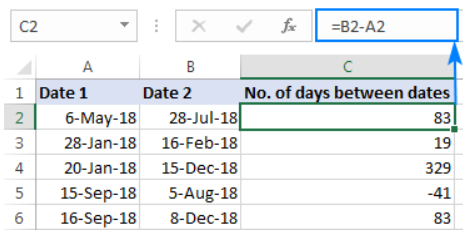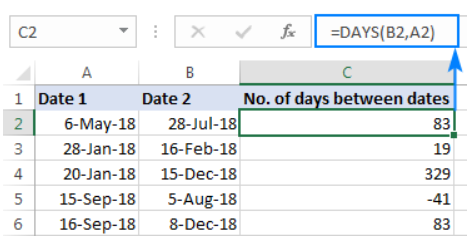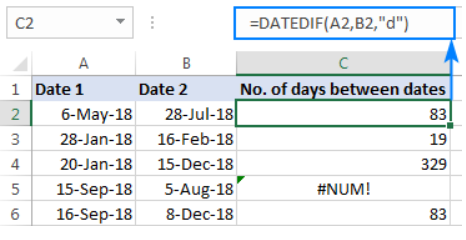# How to Calculate Days Between Two Dates in Excel 2023

Affiliate disclosure: In full transparency – some of the links on our website are affiliate links, if you use them to make a purchase we will earn a commission at no additional cost for you (none whatsoever!).

MS Excel is a magical workbook for professionals. If you go and ask a professional about the importance of excel and the features offered by it, probably you’ll have to take your day off and the list won’t end.

From adding, subtracting, and splitting to finding out the difference between two dates, Excel has everything to offer you can expect from the software.

And today we are going to talk about the ways to calculate days between two dates.

Yes, we will learn Excel date differences whether it’s about days, months, or years!

There are a number of ways we can find out the difference between dates in excel and we will tell you each and every method in the best possible fashion.

But before we could go and learn how to subtract dates in Excel, it’s really important to know why do we even need to do that??

What’s the need to subtract dates in excel?

Well, we have answers to all your queries but in order to figure out those answers, we will have to dive deeper into the tutorial.

## How to Calculate Days Between Two Dates in Excel:

Subtracting dates in Excel and finding out the difference between them is not tough and we will go through all the possible ways for the same but before that, we will figure out the purpose of finding out excel days between dates.

• If you are a student, you need to calculate days between two days while filling any examination form. Because we require to filling out our age in years, months and days.
• If you are a business, you need to subtract days to figure out how many days did your customer take to clear their debt.
• If you have a goal to achieve, you calculate the difference in days to find out the exact number of days you took to achieve the goal and so on.

Apparently, finding out the difference in dates on fingers is pretty old school, that too when you have a large sheet to calculate the dates of. So, how do we exactly calculate the difference between the dates in Excel, let’s find out!

## How To Calculate Days Between Two Dates:

Calculating the days between two dates in Excel is considerably easy. It’s just similar to subtracting two numbers. If you have ever tried your hands on excel sheets, I’m sure you know how to add and subtract in excel.

If you don’t know how to do that, no worries. Simply follow our guide! To calculate days between two dates in Excel, we have multi formulas. One is as simple as subtracting.

Let’s see how to apply those formulas in excel and get the job done quickly.

#### #Frist Formula: =B2-A2

Simply apply =B2-A2, which is a basic formula for subtracting. Excel is smart enough to understand the data you have entered is a date, and it will give you the answer in days. See the below screenshot to understand how this formula works in Excel.#### #Second Formula: =DAYS(B2, A1)

Alternatively, you can use =DAYS(B2, A1). There is nothing different in this formula except the word DAYS used, before the brackets.#### #Third Formula: =DATEDIF(A2, B2, “d”)

=DATEDIF(A2, B2, “d”) works exactly the same and gives the difference in days between two different dates.## How To Calculate Months Between Two Dates:

Calculating the months between two dates is a little complicated. Because it follows two different formulas for finding out the difference between the two dates in the same year and for the dates which fall more than a year apart.

#### # Calculating months between the same year: =MONTH(A1)-MONTH(A2)

If you have two dates of the same year and you want to calculate the months between them, the below formula might help you. However, it’s a pretty simple calculation and can be done on fingers but only then, when you have very limited calculations to be performed.

But when the list is long, putting up the formula in excel is the best choice.

Now coming to the point. Suppose, we have two dates of the same year i.e 6/9/2008 and 6/4/2008 and we have to calculate the months between them, here’s how can you do this.

#### # Calculating months between the dates more than a year apart: =(YEAR(A2)-YEAR(A1))*12+MONTH(A2)-MONTH(A1)

If you have to calculate months between the dates that don’t fall in the same year, apply this formula, =(YEAR(A2)-YEAR(A1))*12+MONTH(A2)-MONTH(A1) and get your job done.

## How to Calculate Years Between Two Dates:

Unlike calculating months, there is no such problem in calculating the years between two dates.

Although we have options to choose between the two formulas we are not limited to use any particular formula in a particular situation.

Confused?? No need. I simply mean we have two formulas to calculate years between two dates.

#### #Frist Formula: =DATEDIF(A2, A1, “Y”)

See the above-mentioned formula and apply it to your excel sheet to calculate the year difference between two dates. Without given much explanation on it.

#### #Second Formula: =YEAR(A2)-YEAR(A1)

It’s an alternative method to calculate the years between two dates. Although the first method is sufficient to help you find out the difference if you want to, you can try out the below-given formula too.

It is also very simple. The result you will get by both the methods is exactly the same.

=YEAR(A2)-YEAR(A1)

Pretty easy, right? That’s how we calculate days between two dates, months, and years!

Most often we assume problems as big but in actual they are too easy to solve. So it’s time for us to wrap up the guide. I hope the guide was of significant help to you!